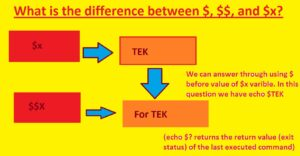Hello, readers welcome to new post. In this post, we will learn What is the difference between \$, \$\$, and \$x. In computer programming, \$, \$\$, and \$x are called variables and used for storing the data. The basic difference between them is that \$ is a scalar variable, \$\$ array variable and \$x is a variable that is used to store both scalar and array data.

In this post, we will discuss different points and solve examples to find the differences. So let get started  What is the difference between \$, \$\$, and \$x?

### What is the difference between \$, \$\$, and \$x?

• The \$x or single dollar is a variable with is a name that stores any value such as integer, string, float, etc. While \$\$x or double dollar is a reference variable that stores value that can access through use of \$ before \$X. These values are known as variable variables in the PHP language.

What is variable Variables?

• These are just variables their names are dynamically made through the use of other variable’s value• We can get an understanding from the above figure.
• \$X stores value “TEK” in form of a string
• After that reference value, \$\$X stores value “for TEK” in form of string type
• There are two ways to access the value of “for TEK”.
• First is the use of reference variables like echo \$\$X
• Second is the value stored in variable \$x in form of the variable name to access “for TEK”. Like echo \$TEK, that will provide output as  for TEK

Now solve example

```Input : \$x = "Geeks";
\$\$x = for TEK;
echo "\$x ";
echo "\$\$x;";
echo \$TEK;
Output : TEK
for TEK
for TEK

Input : \$x = "THE";
\$\$x = "ENGINEERING";
echo "\$x KNOWLEDGE " . \$THE;
Output : THE ENGINEERING KNOWLEDGE
Now solve example to understand \$ and \$\$

```
`<?php`
`// Declare variable and initialize it`
`\$x` `= "TEK";    `
`// Reference variable`
`\$``\$x` `= "TEKforTEK";`
`// Display value of x`
`echo` `\$x` `. "\n";`
`// Display value of \$\$x (\$TEK)`
`echo` `\$``\$x` `. "\n";`
`// Display value of \$TEK`
`echo` `"``\$TEK``";`
`?>`

Output:

```TEK
TEKforTEK
TEKforTEK

```
Symbol PHP jQuery Bash
\$ Used to denote a variable Alias for jQuery() function Used to reference the value of a variable
\$\$ Used to create a variable variable N/A Used to reference the process ID of the current shell
\$x Not recommended for variable naming N/A Used to reference the value of a variable
```That is all about the difference between \$, \$\$, and \$x? all point has explained if you have any question ask here

```